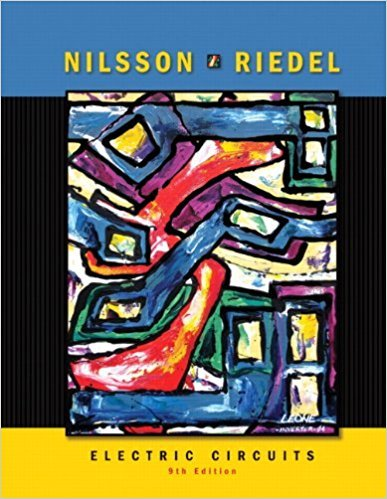×
×

# Assume the voltage divider in Fig. P3.16 has been constructed from 1 W resistors. WhatISBN: 9780136114994 391

## Solution for problem 3.17 Chapter 3

Electric Circuits | 9th Edition

• Textbook Solutions
• 2901 Step-by-step solutions solved by professors and subject experts
• Get 24/7 help from StudySoup virtual teaching assistantsElectric Circuits | 9th Edition

4 5 1 397 Reviews
18
4
Problem 3.17

Assume the voltage divider in Fig. P3.16 has been constructed from 1 W resistors. What is the smallest resistor from Appendix H that can be used as RL before one of the resistors in the divider is operating at its dissipation limit?

Step-by-Step Solution:
Step 1 of 3

Day 5 ­fprintf­This function is used to format output ­anything written in single parentheses that becomes a string of text is a string value, or a numerical value see picture below to see the syntax). Example: >> y=input('Enter a number') Enter a number Or >> z = input('Enter Text:','s') Enter Text: ­Syntax:fprintf(format_string,...

Step 2 of 3

Step 3 of 3

##### ISBN: 9780136114994

The answer to “Assume the voltage divider in Fig. P3.16 has been constructed from 1 W resistors. What is the smallest resistor from Appendix H that can be used as RL before one of the resistors in the divider is operating at its dissipation limit?” is broken down into a number of easy to follow steps, and 42 words. Electric Circuits was written by and is associated to the ISBN: 9780136114994. The full step-by-step solution to problem: 3.17 from chapter: 3 was answered by , our top Engineering and Tech solution expert on 03/13/18, 07:50PM. This textbook survival guide was created for the textbook: Electric Circuits, edition: 9. Since the solution to 3.17 from 3 chapter was answered, more than 217 students have viewed the full step-by-step answer. This full solution covers the following key subjects: . This expansive textbook survival guide covers 18 chapters, and 1109 solutions.

Unlock Textbook Solution